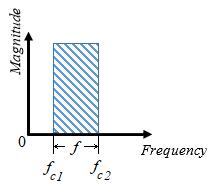# Second-Order Filter

Discrete-time or continuous-time low-pass, high-pass, band-pass, or band-stop second-order filter

•Libraries:
Simscape / Electrical / Control / General Control

## Description

The Second-Order Filter block implements different types of second-order filters. Filters are useful for attenuating noise in measurement signals.

The block provides these filter types:

• Low pass — Allows signals, $f$, only in the range of frequencies below the cutoff frequency, ${f}_{c}$, to pass.

• High pass — Allows signals, $f$, only in the range of frequencies above the cutoff frequency, ${f}_{c}$, to pass.

• Band pass — Allows signals, $f$, only in the range of frequencies between two cutoff frequencies, ${f}_{c1}$ and ${f}_{c2}$, to pass.

• Band stop — Prevents signals, $f$, only in the range of frequencies between two cutoff frequencies, ${f}_{c1}$ and ${f}_{c2}$, from passing.

Filter TypeFrequency Range, $f$
Low-Pass$f<{f}_{c}$
High-Pass$f>{f}_{c}$
Band-Pass${f}_{c1}
Band-Stop${f}_{c1}

### Equations

The second order derivative state equation for the filter is:

`$\frac{{d}^{2}x}{d{t}^{2}}=u-2\zeta {\omega }_{n}\frac{dx}{dt}-{\omega }_{n}^{2}x$`

Where:

• x is the filter internal state.

• u is the filter input.

• ωn is the filter natural frequency.

• ζ is the filter damping factor.

For each filter type, the table maps the block output, $y\left(x\right)$, as a function of the internal state of the filter, to the s-domain transfer function, $G\left(s\right)$.

Filter TypeOutput, $y\left(x\right)$Transfer Function, $G\left(s\right)$
Low-Pass${\omega }_{n}^{2}x$$\frac{{\omega }_{n}^{2}}{{s}^{2}+2\zeta {\omega }_{n}s+{\omega }_{n}^{2}}$
High-Pass$\frac{{d}^{2}x}{d{t}^{2}}$$\frac{{s}^{2}}{{s}^{2}+2\zeta {\omega }_{n}s+{\omega }_{n}^{2}}$
Band-Pass$2\zeta {\omega }_{n}\frac{dx}{dt}$$\frac{2\zeta {\omega }_{n}s}{{s}^{2}+2\zeta {\omega }_{n}s+{\omega }_{n}^{2}}$
Band-Stop$\frac{{d}^{2}x}{d{t}^{2}}+x$$\frac{{s}^{2}+{\omega }_{n}^{2}}{{s}^{2}+2\zeta {\omega }_{n}s+{\omega }_{n}^{2}}$

For Initialization:

`$\stackrel{˙}{x}\left(0\right)={\frac{dx}{dt}|}_{t=0}$`

`$u\left(0\right)={u}_{1}\left(0\right)+{u}_{2}\left(0\right)$`

`${u}_{1}\left(0\right)={A}_{0}{e}^{j{\phi }_{0}}$`

`${u}_{2}\left(0\right)={b}_{0}{e}^{j\frac{\pi }{2}}$`

Where:

• $x\left(0\right)$ is the initial state of the filter.

• $u\left(0\right)$ is the initial input to the filter.

• ${u}_{1}\left(0\right)$ is the AC component of the steady-state initial input.

• ${A}_{0}$ is the initial amplitude.

• ${\phi }_{0}$ is the initial phase.

• ${u}_{2}\left(0\right)$ is the DC component of the steady-state initial input.

• ${b}_{0}$ is the initial bias.

In the s-domain $s=j{\omega }_{0}$. Therefore, for the initial frequency, ${\omega }_{0}$:

`$\stackrel{˙}{x}\left(0\right)=Im\left(\frac{j{\omega }_{0}{u}_{1}\left(0\right)}{-{\omega }_{0}^{2}+j{\omega }_{0}2\zeta {\omega }_{n}+{\omega }_{n}^{2}}\right).$`

`$x\left(0\right)=Im\left(\frac{\stackrel{˙}{x}\left(0\right){\omega }_{n}^{2}}{j{\omega }_{0}}+{u}_{2}\left(0\right)\right)$`

## Ports

### Input

expand all

Filter input.

Data Types: `single` | `double`

### Output

expand all

Filtered output.

Data Types: `single` | `double`

## Parameters

expand all

### Main

Type of second-order filter.

Natural frequency, in Hz.

### Initial Conditions

Damping factor of the filter.

Time between consecutive block executions. During execution, the block produces outputs and, if appropriate, updates its internal state. For more information, see What Is Sample Time? and Specify Sample Time.

For inherited discrete-time operation, set this parameter to `-1`. For discrete-time operation, set this parameter to a positive integer. For continuous-time operation, set this parameter to `0`.

If this block is in a masked subsystem or a variant subsystem that supports switching between continuous operation and discrete operation, promote this parameter to ensure correct switching between the continuous and discrete implementations of the block. For more information, see Promote Block Parameters on a Mask.

Amplitude at the start of simulation.

Phase, in rad, at the start of simulation.

Frequency, in Hz, at the start of simulation.

Bias at the start of simulation.

 Agarwal, A. and Lang, J. H. Foundations of Analog and Digital Electronic Circuits. New York: Elsevier, 2005.